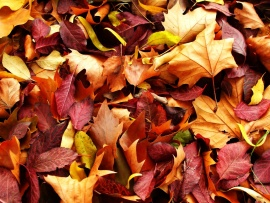WE USE COOKIES           ** FACEBOOK PAGE
The colorful time of Autumn is seen on this Fall wallpaper. Brightly colored leaves make up the blanket of this wallpaper. Before the 16th century, 'harvest' was the term used to refer to the Autumn season. Fall is also used as a term for Autumn, but is mostly a North American English term.## Download Autumn Leaves wallpaper | Leave a Comment

### function roundNumber(b,c){var a=Math.round(b*Math.pow(10,c))/Math.pow(10,c);return a}function resPrints(){var b=screen.width;var c=screen.height;var a=roundNumber(b/c,2);var d="Your display Resolution: <strong> "+b+" x "+c+" </strong> | Aspect Ratio:<strong> ";if(a==1.25||a==1.33){d+="Standard 4:3"}else{if(a==1.6||a==1.67){d+="Wide 8:5"}else{if(a==1.78){d+="HD 16:9"}else{if(a==1.76){d+="Mobile PSP"}else{if(a==0.75||(a==1.33&&b<=640)){d+="Mobile VGA"}else{if(a==0.6||(a==1.67&&b<=800)){d+="Mobile WVGA"}else{if(a==0.67||(a==1.5&&b==480)){d+="Mobile iPhone"}else{if(a==0.8){d+="Mobile Phone"}else{d="Unknown"}}}}}}}}d+="</strong>";document.write(d)}; resPrints();

Wallpaper details:
Added: Monday, 04 October 2010 16:01
Downloads: 64922
Sub Categories:
License: Free for personal wallpaper use only